# Latent projection predictive feature selection

## Introduction

This vignette shows how to use the latent projection predictive feature selection from Catalina, Bürkner, and Vehtari (2021) in projpred. We recommend to read the main vignette first, as the latent-projection vignette presented here will skip some of the details explained in the main vignette.

### General idea

The response families used in GLMs (and in GLMMs, GAMs, and GAMMs) may be termed exponential dispersion (ED) families 1. For a response family that is not an ED family, the Kullback-Leibler (KL) divergence minimization problem is often not easy to solve analytically2. In order to bypass this issue, the latent projection solves the KL minimization problem in the predictive space of the latent predictors3 instead of in the predictive space of the original response values.

To this end, the latent predictor is assumed to have a Gaussian distribution, since it (i) constitutes a combination of predictor data and regression parameters which is often linear (in the parameters, but—less—often also in the predictor data) or at least additive (across the predictor terms) and (ii) has the complete real line as support. Furthermore, the Gaussian distribution has the highest differential entropy among all distributions with two finite moments and with the real line as support (see, e.g., Cover and Thomas 1991). In some cases, e.g., for the probit link, the Gaussian distribution is even part of the original statistical model. In case of the logit link, the Gaussian distribution with a standard deviation of 1.6 approximates the logistic distribution (with a scale parameter of 1).

The assumption of a Gaussian distribution for the latent predictors makes things a lot easier because it allows us to make use of projpred’s traditional projection.

As illustrated by the Poisson example below, the latent projection can not only be used for families not supported by projpred’s traditional projection, but it can also be beneficial for families supported by it.

### Implementation

To use the latent projection in projpred, the new argument latent of extend_family() needs to be set to TRUE. Since extend_family() is called by init_refmodel() which in turn is called by get_refmodel() (more precisely, by the get_refmodel() methods) which in turn is called at the beginning of the top-level functions project(), varsel(), and cv_varsel(), it is possible to pass latent = TRUE from such a top-level function down to extend_family() via the ellipsis (...). However, for the latent projection, we recommend to define the reference model object of class refmodel explicitly (as illustrated in the examples below) to avoid repetitions4.

After performing the projection (either as a stand-alone feature via project() or embedded in a variable selection via varsel() or cv_varsel()), the post-processing (e.g., the calculation of the performance statistics in summary.vsel()) can be performed on the original response scale. For this, extend_family() has gained several new arguments accepting R functions responsible for the inverse-link transformation from latent scale to response scale (latent_ilink), for the calculation of log-likelihood values on response scale (latent_ll_oscale), and for drawing from the (posterior-projection) predictive distribution on response scale (latent_ppd_oscale). For some families, these arguments have internal defaults implemented natively in projpred. These families are listed in the main vignette (section “Supported types of models”). For all other families, projpred either tries to infer a reasonable function internally (in case of latent_ilink) or uses a dummy function returning only NAs (in case of latent_ll_oscale and latent_ppd_oscale), unless the user supplies custom functions to these arguments. When creating a reference model object for a family of the latter category (i.e., lacking full response-scale support by default), projpred will throw messages stating whether (and which) features will be unavailable unless at least some of these arguments are provided by the user. Again, the ellipsis (...) can be used to pass these arguments from a top-level function such as cv_varsel() down to extend_family(). In the post-processing functions, response-scale analyses can usually be deactivated by setting the new argument resp_oscale to FALSE, with the exception of predict.refmodel() and proj_linpred() where the existing arguments type and transform serve this purpose (see the documentation).

Apart from the arguments mentioned above, extend_family() has also gained a new argument latent_y_unqs whose purpose is described in the documentation.

While the latent projection is an approximate solution to the KL divergence minimization problem in the original response space5, the augmented-data projection gives the exact6 solution for some non-ED families, namely those where the response distribution has finite support. However, the augmented-data projection comes with a higher runtime than the latent projection. The families supported by projpred’s augmented-data projection are also listed in the main vignette (again section “Supported types of models”).

## Example: Poisson distribution

In this example, we will illustrate that in case of a family supported by projpred’s traditional projection (here the Poisson distribution), the latent projection can improve runtime and results of the variable selection compared to projpred’s traditional projection, at least if the L1 search is used (see argument method of varsel() and cv_varsel()).

### Data

First, we generate a training and a test dataset with a Poisson-distributed response:

# Number of observations in the training dataset (= number of observations in
# the test dataset):
N <- 71
# Data-generating function:
sim_poiss <- function(nobs = 2 * N, ncon = 10, ncats = 4, nnoise = 39) {
# Regression coefficients for continuous predictors:
coefs_con <- rnorm(ncon)
# Continuous predictors:
dat_sim <- matrix(rnorm(nobs * ncon), ncol = ncon)
# Start linear predictor:
linpred <- 2.1 + dat_sim %*% coefs_con

# Categorical predictor:
dat_sim <- data.frame(
x = dat_sim,
xcat = gl(n = ncats, k = nobs %/% ncats, length = nobs,
labels = paste0("cat", seq_len(ncats)))
)
# Regression coefficients for the categorical predictor:
coefs_cat <- rnorm(ncats)
# Continue linear predictor:
linpred <- linpred + coefs_cat[dat_sim$xcat] # Noise predictors: dat_sim <- data.frame( dat_sim, xn = matrix(rnorm(nobs * nnoise), ncol = nnoise) ) # Poisson response, using the log link (i.e., exp() as inverse link): dat_sim$y <- rpois(nobs, lambda = exp(linpred))
# Shuffle order of observations:
dat_sim <- dat_sim[sample.int(nobs), , drop = FALSE]
# Drop the shuffled original row names:
rownames(dat_sim) <- NULL
return(dat_sim)
}
# Generate data:
set.seed(300417)
dat_poiss <- sim_poiss()
dat_poiss_test <- tail(dat_poiss, N)

### Reference model

Next, we fit the reference model that we consider as the best model in terms of predictive performance that we can construct (here, we assume that we don’t know about the true data-generating process even though the dataset was simulated):

library(rstanarm)
# Number of regression coefficients:
( D <- sum(grepl("^x", names(dat_poiss_train))) )
 50
# Prior guess for the number of relevant (i.e., non-zero) regression
# coefficients:
p0 <- 10
# Prior guess for the overall magnitude of the response values, see Table 1 of
# Piironen and Vehtari (2017, DOI: 10.1214/17-EJS1337SI):
mu_prior <- 100
# Hyperprior scale for tau, the global shrinkage parameter:
tau0 <- p0 / (D - p0) / sqrt(mu_prior) / sqrt(N)
# Set this manually if desired:
ncores <- parallel::detectCores(logical = FALSE)
### Only for technical reasons in this vignette (you can omit this when running
### the code yourself):
ncores <- min(ncores, 2L)
###
options(mc.cores = ncores)
refm_fml <- as.formula(paste("y", "~", paste(
grep("^x", names(dat_poiss_train), value = TRUE),
collapse = " + "
)))
refm_fit_poiss <- stan_glm(
formula = refm_fml,
family = poisson(),
data = dat_poiss_train,
prior = hs(global_scale = tau0, slab_df = 100, slab_scale = 1),
### Only for the sake of speed (not recommended in general):
chains = 2, iter = 1000,
###
QR = TRUE, refresh = 0
)

### Variable selection using the latent projection

Within projpred, we define the reference model object explicitly and set latent = TRUE in the corresponding get_refmodel() call (see section “Implementation”) so that the latent projection is used in downstream functions. Since we have a hold-out test dataset available, we can use varsel() with argument d_test instead of cv_varsel(). Furthermore, we measure the runtime to be able to compare it to the traditional projection’s later:

library(projpred)
d_test_lat_poiss <- list(
data = dat_poiss_test,
offset = rep(0, nrow(dat_poiss_test)),
weights = rep(1, nrow(dat_poiss_test)),
### Here, we are not interested in latent-scale post-processing, so we can set
### element y to a vector of NAs:
y = rep(NA, nrow(dat_poiss_test)),
###
y_oscale = dat_poiss_test$y ) refm_poiss <- get_refmodel(refm_fit_poiss, latent = TRUE) Since <refmodel>$dis will consist of only NAs, downstream analyses based on this reference model object won't be able to use log predictive density (LPD) values on latent scale. Furthermore, proj_predict() won't be able to draw from the latent Gaussian distribution.
time_lat <- system.time(vs_lat <- varsel(
refm_poiss,
d_test = d_test_lat_poiss,
### Only for demonstrating an issue with the traditional projection in the
### next step (not recommended in general):
method = "L1",
###
### Only for the sake of speed (not recommended in general):
nclusters_pred = 20,
###
nterms_max = 14,
### In interactive use, we recommend not to deactivate the verbose mode:
verbose = FALSE,
###
### For comparability with varsel() based on the traditional projection:
seed = 95930
###
))
print(time_lat)
   user  system elapsed
1.638   0.016   1.654 

The message telling that <refmodel>$dis consists of only NAs will not concern us here because we will only be focusing on response-scale post-processing. In order to decide for a submodel size, we first inspect the plot() results: ( gg_lat <- plot(vs_lat, stats = "mlpd", deltas = TRUE) )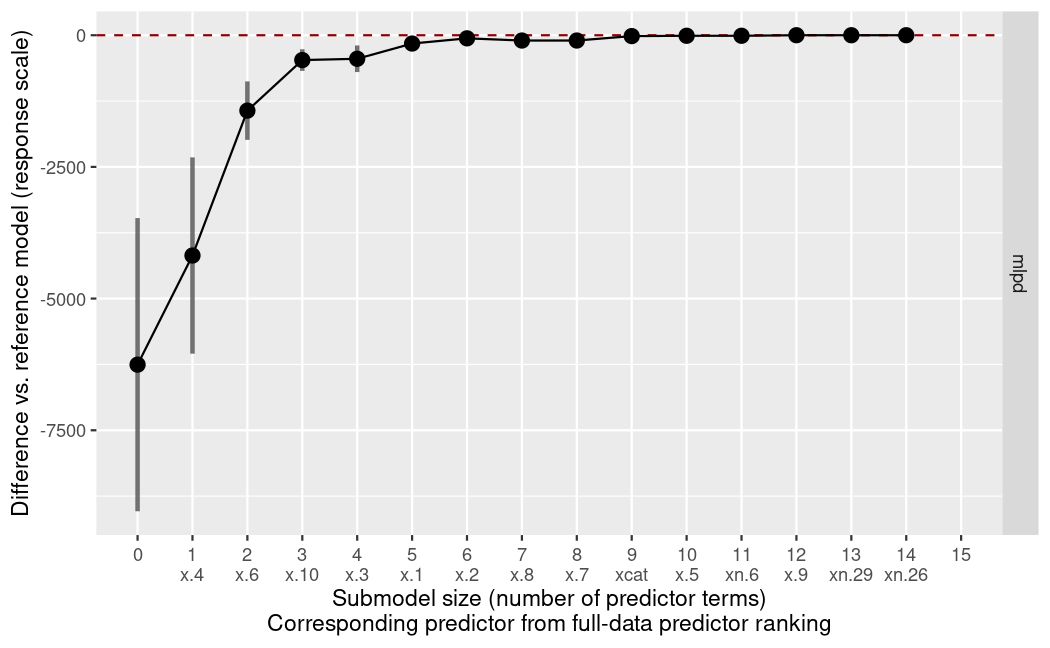Although the submodels’ MLPDs seem to be very close to the reference model’s MLPD from a submodel size of 6 on, a zoomed plot reveals that there is still some discrepancy at sizes 6 to 11 and that size 12 would be a better choice (further down below in the summary() output, we will also see that on absolute scale, the discrepancy at sizes 6 to 11 is not negligible): gg_lat + ggplot2::coord_cartesian(ylim = c(-10, 0.05))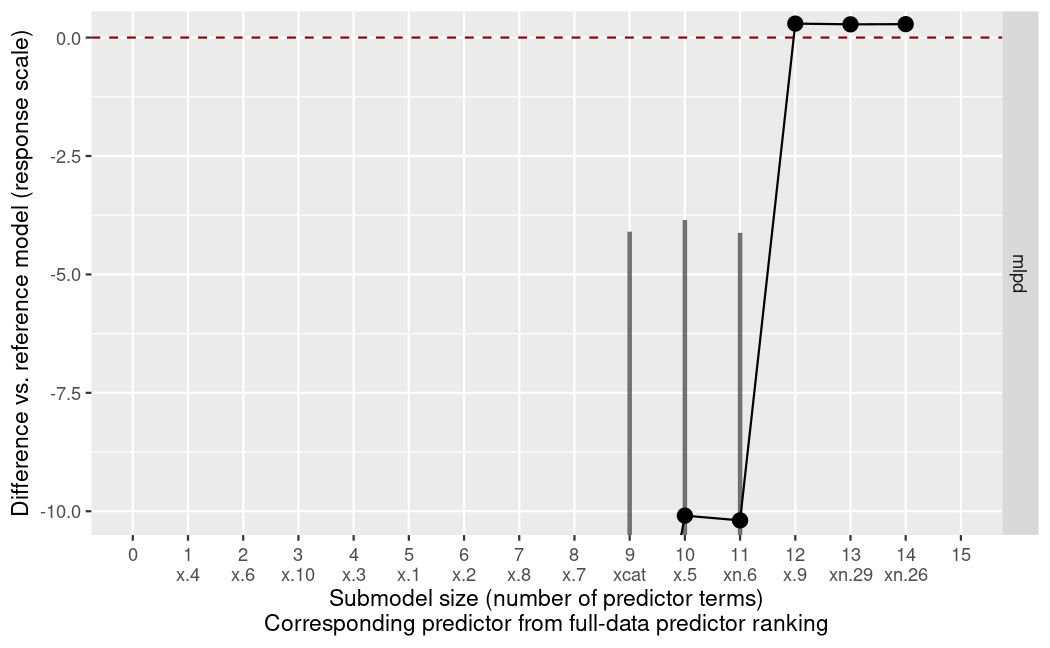Thus, we decide for a submodel size of 12: size_decided_lat <- 12 This is also the size that suggest_size() would suggest: suggest_size(vs_lat, stat = "mlpd")  12 To obtain the results from the varsel() run in tabular form, we call summary.vsel(): smmry_lat <- summary(vs_lat, stats = "mlpd", type = c("mean", "lower", "upper", "diff")) print(smmry_lat, digits = 2) ------ Response-scale family: Family: poisson Link function: log ------ Latent-scale family: Family: gaussian Link function: identity ------ Formula: .y ~ x.1 + x.2 + x.3 + x.4 + x.5 + x.6 + x.7 + x.8 + x.9 + x.10 + xcat + xn.1 + xn.2 + xn.3 + xn.4 + xn.5 + xn.6 + xn.7 + xn.8 + xn.9 + xn.10 + xn.11 + xn.12 + xn.13 + xn.14 + xn.15 + xn.16 + xn.17 + xn.18 + xn.19 + xn.20 + xn.21 + xn.22 + xn.23 + xn.24 + xn.25 + xn.26 + xn.27 + xn.28 + xn.29 + xn.30 + xn.31 + xn.32 + xn.33 + xn.34 + xn.35 + xn.36 + xn.37 + xn.38 + xn.39 Observations (training set): 71 Observations (test set): 71 Projection method: latent Search method: L1 Maximum submodel size for the search: 14 Number of projected draws in the search: 1 (from clustered projection) Number of projected draws in the performance evaluation: 20 (from clustered projection) Performance evaluation summary (response scale) with deltas = FALSE and cumulate = FALSE: size solution_terms cv_proportions_diag mlpd lower upper diff 0 <NA> NA -6257.6 -9041.1 -3474.0 -6254.36 1 x.4 NA -4185.6 -6049.0 -2322.2 -4182.39 2 x.6 NA -1434.7 -1989.6 -879.8 -1431.46 3 x.10 NA -474.7 -679.9 -269.4 -471.45 4 x.3 NA -450.2 -703.2 -197.1 -446.98 5 x.1 NA -161.0 -244.8 -77.2 -157.77 6 x.2 NA -62.1 -90.6 -33.6 -58.89 7 x.8 NA -103.8 -158.4 -49.1 -100.58 8 x.7 NA -103.6 -158.5 -48.8 -100.42 9 xcat NA -19.6 -31.9 -7.2 -16.35 10 x.5 NA -13.3 -19.7 -6.9 -10.09 11 xn.6 NA -13.4 -19.6 -7.2 -10.19 12 x.9 NA -2.9 -3.2 -2.6 0.30 13 xn.29 NA -2.9 -3.2 -2.6 0.28 14 xn.26 NA -2.9 -3.2 -2.6 0.28 On absolute scale (column mlpd), we see that submodel size 11 leads to an MLPD of -13.4, i.e., a geometric mean predictive density (GMPD; due to the discrete response family, the “density” values are probabilities here, so we will report the GMPD as percentage) of exp(-13.4) which is ca. 2e-04 % whereas size 12 leads to a GMPD of ca. 5.5 %. This is a considerable improvement from size 11 to size 12, so another justification for size 12. (Size 13 would have resulted in about the same GMPD as size 12.) In the predictor ranking up to the selected size of 12, we can see that apart from the noise term xn.6, projpred has correctly selected the truly relevant predictors first and only then the noise predictors. We can see this more clearly using the following code: rk_lat <- ranking(vs_lat) ( predictors_final_lat <- head(rk_lat[["fulldata"]], size_decided_lat) )   "x.4" "x.6" "x.10" "x.3" "x.1" "x.2" "x.8" "x.7" "xcat" "x.5"  "xn.6" "x.9"  We will skip post-selection inference here (see the main vignette for a demonstration of post-selection inference), but note that proj_predict() has gained a new argument resp_oscale and that analogous response-scale functionality is available in proj_linpred() (argument transform) and predict.refmodel() (argument type). ### Variable selection using the traditional projection We will now look at what projpred’s traditional projection would have given: d_test_trad_poiss <- d_test_lat_poiss d_test_trad_poiss$y <- d_test_trad_poiss$y_oscale d_test_trad_poiss$y_oscale <- NULL
refm_fit_poiss,
### Only for demonstrating an issue with the traditional projection (not
### recommended in general):
method = "L1",
###
### Only for the sake of speed (not recommended in general):
nclusters_pred = 20,
###
nterms_max = 14,
### In interactive use, we recommend not to deactivate the verbose mode:
verbose = FALSE,
###
### For comparability with varsel() based on the latent projection:
seed = 95930
###
))
print(time_trad)
   user  system elapsed
2.533   0.004   2.536 
( gg_trad <- plot(vs_trad, stats = "mlpd", deltas = TRUE) )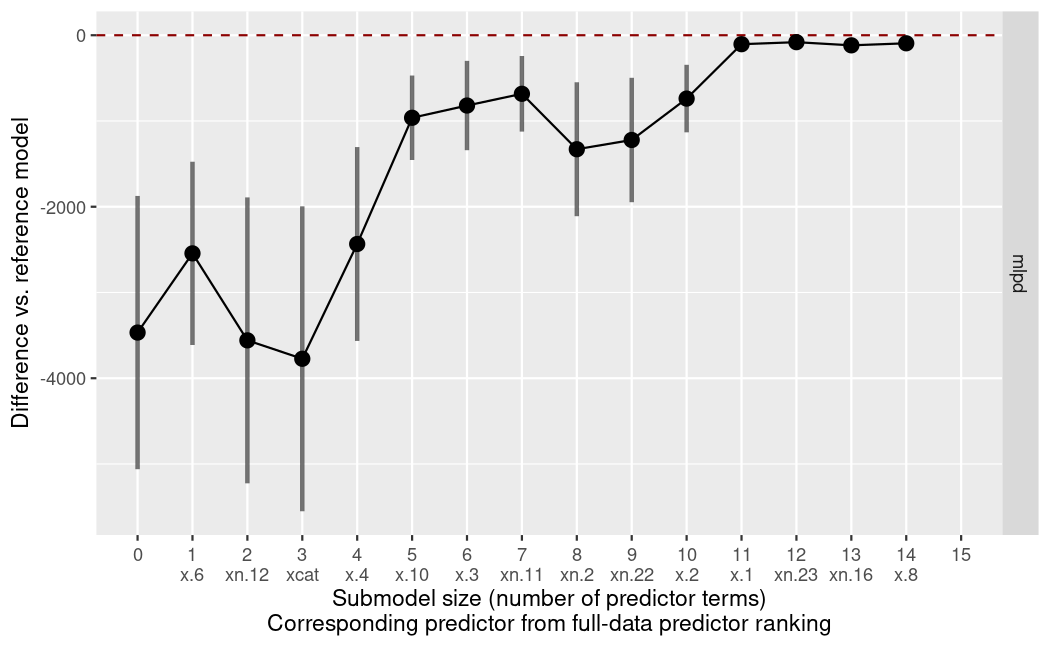smmry_trad <- summary(vs_trad, stats = "mlpd",
type = c("mean", "lower", "upper", "diff"))
print(smmry_trad, digits = 2)

Family: poisson

Formula: y ~ x.1 + x.2 + x.3 + x.4 + x.5 + x.6 + x.7 + x.8 + x.9 + x.10 +
xcat + xn.1 + xn.2 + xn.3 + xn.4 + xn.5 + xn.6 + xn.7 + xn.8 +
xn.9 + xn.10 + xn.11 + xn.12 + xn.13 + xn.14 + xn.15 + xn.16 +
xn.17 + xn.18 + xn.19 + xn.20 + xn.21 + xn.22 + xn.23 + xn.24 +
xn.25 + xn.26 + xn.27 + xn.28 + xn.29 + xn.30 + xn.31 + xn.32 +
xn.33 + xn.34 + xn.35 + xn.36 + xn.37 + xn.38 + xn.39
Observations (training set): 71
Observations (test set): 71
Search method: L1
Maximum submodel size for the search: 14
Number of projected draws in the search: 1 (from clustered projection)
Number of projected draws in the performance evaluation: 20 (from clustered projection)

Performance evaluation summary with deltas = FALSE and cumulate = FALSE:
size solution_terms cv_proportions_diag  mlpd lower upper  diff
0           <NA>                  NA -3471 -5064 -1877 -3467
1            x.6                  NA -2547 -3616 -1478 -2544
2          xn.12                  NA -3562 -5229 -1894 -3558
3           xcat                  NA -3776 -5555 -1998 -3773
4            x.4                  NA -2438 -3568 -1307 -2435
5           x.10                  NA  -966 -1459  -473  -963
6            x.3                  NA  -823 -1344  -302  -820
7          xn.11                  NA  -686 -1127  -246  -683
8           xn.2                  NA -1333 -2114  -551 -1329
9          xn.22                  NA -1225 -1950  -499 -1222
10            x.2                  NA  -742 -1136  -348  -739
11            x.1                  NA  -108  -145   -71  -105
12          xn.23                  NA   -84  -118   -51   -81
13          xn.16                  NA  -121  -188   -54  -118
14            x.8                  NA   -97  -144   -51   -94

As these results show, the traditional projection takes longer than the latent projection, although the difference is rather small on absolute scale (which is due to the fact that the L1 search is already quite fast). More importantly however, the predictive performance plot is much more unstable and the predictor ranking contains several noise terms before truly relevant ones.

### Conclusion

In conclusion, this example showed that the latent projection can be advantageous also for families supported by projpred’s traditional projection by improving the runtime and the stability of the variable selection, eventually also leading to better variable selection results.

An important point is that we have used the L1 search here. In case of the latent projection, a forward search would have given only slightly different results (in particular, a slightly smoother predictive performance plot). However, in case of the traditional projection, a forward search would have given markedly better results (in particular, the predictive performance plot would have been much smoother and all of the noise terms would have been selected after the truly relevant ones). Thus, the conclusions made for the L1 search here cannot be transmitted easily to the forward search.

## Example: Negative binomial distribution

In this example, we will illustrate the latent projection in case of the negative binomial family (more precisely, we are using rstanarm::neg_binomial_2() here) which is a family that is not supported by projpred’s traditional projection7.

### Data

We will re-use the data generated above in the Poisson example.

### Reference model

We now fit a reference model with the negative binomial distribution as response family. For the sake of simplicity, we won’t adjust tau0 to this new family, but in a real-world example, such an adjustment would be necessary. However, since Table 1 of Piironen and Vehtari (2017) doesn’t list the negative binomial distribution, this would first require a manual derivation of the pseudo-variance $$\tilde{\sigma}^2$$.

refm_fit_nebin <- stan_glm(
formula = refm_fml,
family = neg_binomial_2(),
data = dat_poiss_train,
prior = hs(global_scale = tau0, slab_df = 100, slab_scale = 1),
### Only for the sake of speed (not recommended in general):
chains = 2, iter = 1000,
###
QR = TRUE, refresh = 0
)

### Variable selection using the latent projection

To request the latent projection with latent = TRUE, we now need to specify more arguments (latent_ll_oscale and latent_ppd_oscale) which will be passed to extend_family()8:

refm_prec <- as.matrix(refm_fit_nebin)[, "reciprocal_dispersion", drop = FALSE]
latent_ll_oscale_nebin <- function(ilpreds, y_oscale,
wobs = rep(1, length(y_oscale)), cl_ref,
wdraws_ref = rep(1, length(cl_ref))) {
y_oscale_mat <- matrix(y_oscale, nrow = nrow(ilpreds), ncol = ncol(ilpreds),
byrow = TRUE)
wobs_mat <- matrix(wobs, nrow = nrow(ilpreds), ncol = ncol(ilpreds),
byrow = TRUE)
refm_prec_agg <- cl_agg(refm_prec, cl = cl_ref, wdraws = wdraws_ref)
ll_unw <- dnbinom(y_oscale_mat, size = refm_prec_agg, mu = ilpreds, log = TRUE)
return(wobs_mat * ll_unw)
}
latent_ppd_oscale_nebin <- function(ilpreds_resamp, wobs, cl_ref,
wdraws_ref = rep(1, length(cl_ref)),
idxs_prjdraws) {
refm_prec_agg <- cl_agg(refm_prec, cl = cl_ref, wdraws = wdraws_ref)
refm_prec_agg_resamp <- refm_prec_agg[idxs_prjdraws, , drop = FALSE]
ppd <- rnbinom(prod(dim(ilpreds_resamp)), size = refm_prec_agg_resamp,
mu = ilpreds_resamp)
ppd <- matrix(ppd, nrow = nrow(ilpreds_resamp), ncol = ncol(ilpreds_resamp))
return(ppd)
}
refm_nebin <- get_refmodel(refm_fit_nebin, latent = TRUE,
latent_ll_oscale = latent_ll_oscale_nebin,
latent_ppd_oscale = latent_ppd_oscale_nebin)
Defining latent_ilink as a function which calls family$linkinv, but there is no guarantee that this will work for all families. If relying on family$linkinv is not appropriate or if this raises an error in downstream functions, supply a custom latent_ilink function (which is also allowed to return only NAs if response-scale post-processing is not needed).
Since <refmodel>$dis will consist of only NAs, downstream analyses based on this reference model object won't be able to use log predictive density (LPD) values on latent scale. Furthermore, proj_predict() won't be able to draw from the latent Gaussian distribution. vs_nebin <- varsel( refm_nebin, d_test = d_test_lat_poiss, ### Only for the sake of speed (not recommended in general): method = "L1", nclusters_pred = 20, ### nterms_max = 14, ### In interactive use, we recommend not to deactivate the verbose mode: verbose = FALSE ### ) Again, the message telling that <refmodel>$dis consists of only NAs will not concern us here because we will only be focusing on response-scale post-processing. The message concerning latent_ilink can be safely ignored here (the internal default based on family\$linkinv works correctly in this case).

Again, we first inspect the plot() results to decide for a submodel size:

( gg_nebin <- plot(vs_nebin, stats = "mlpd", deltas = TRUE) )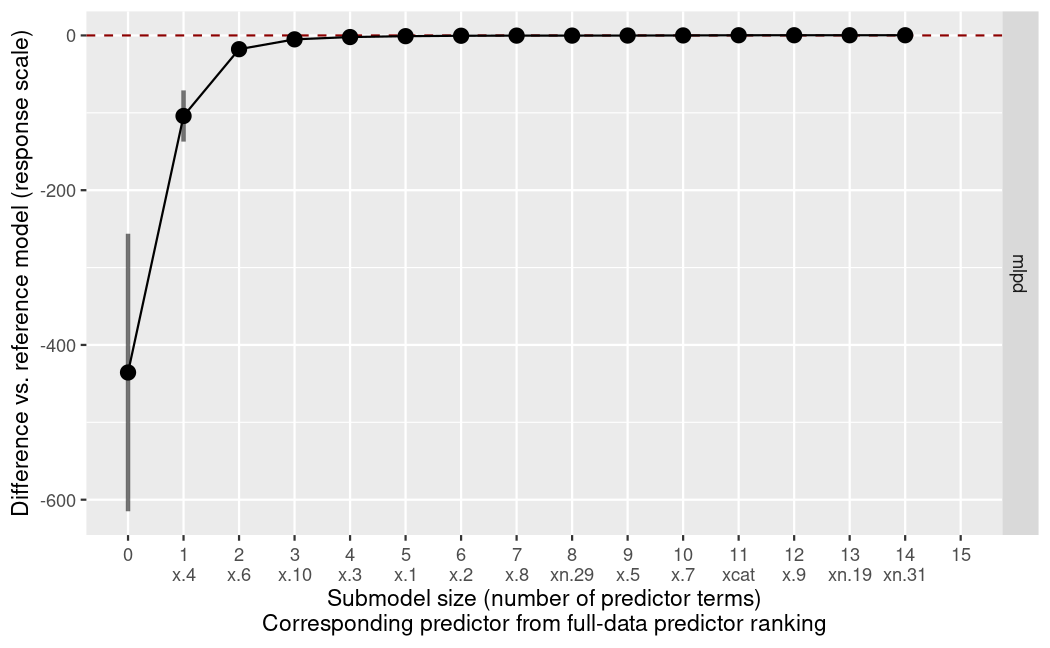Again, a zoomed plot is more helpful:

gg_nebin + ggplot2::coord_cartesian(ylim = c(-2.5, 0.25))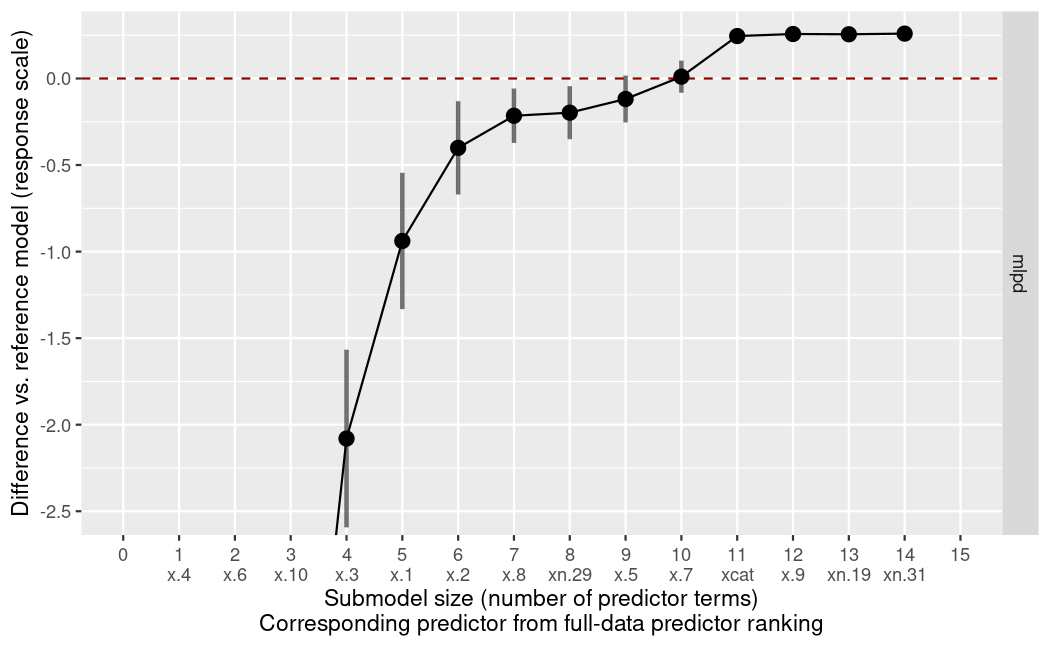Although the submodels’ MLPDs approach the reference model’s already from a submodel size of 9 on (taking into account the uncertainty bars), the curve levels off only from a submodel size of 11 on. For a more informed decision, the GMPD could be taken into account again, but for the sake of brevity, we will simply decide for a submodel size of 11 here:

size_decided_nebin <- 11

This is not the size that suggest_size() would suggest, but as mentioned in the main vignette and in the documentation, suggest_size() provides only a quite heuristic decision (so we stick with our manual decision here):

suggest_size(vs_nebin, stat = "mlpd")
 9

Again, we call summary.vsel() to obtain the results from the varsel() run in tabular form:

smmry_nebin <- summary(vs_nebin, stats = "mlpd",
type = c("mean", "lower", "upper", "diff"))
print(smmry_nebin, digits = 2)
------
Response-scale family:

Family: neg_binomial_2

------
Latent-scale family:

Family: gaussian

------
Formula: .y ~ x.1 + x.2 + x.3 + x.4 + x.5 + x.6 + x.7 + x.8 + x.9 + x.10 +
xcat + xn.1 + xn.2 + xn.3 + xn.4 + xn.5 + xn.6 + xn.7 + xn.8 +
xn.9 + xn.10 + xn.11 + xn.12 + xn.13 + xn.14 + xn.15 + xn.16 +
xn.17 + xn.18 + xn.19 + xn.20 + xn.21 + xn.22 + xn.23 + xn.24 +
xn.25 + xn.26 + xn.27 + xn.28 + xn.29 + xn.30 + xn.31 + xn.32 +
xn.33 + xn.34 + xn.35 + xn.36 + xn.37 + xn.38 + xn.39
Observations (training set): 71
Observations (test set): 71
Projection method: latent
Search method: L1
Maximum submodel size for the search: 14
Number of projected draws in the search: 1 (from clustered projection)
Number of projected draws in the performance evaluation: 20 (from clustered projection)

Performance evaluation summary (response scale) with deltas = FALSE and cumulate = FALSE:
size solution_terms cv_proportions_diag   mlpd  lower  upper    diff
0           <NA>                  NA -439.3 -618.8 -259.7 -435.61
1            x.4                  NA -107.8 -141.1  -74.4 -104.09
2            x.6                  NA  -21.4  -28.2  -14.7  -17.77
3           x.10                  NA   -8.7  -10.0   -7.5   -5.06
4            x.3                  NA   -5.8   -6.5   -5.0   -2.08
5            x.1                  NA   -4.6   -5.2   -4.0   -0.94
6            x.2                  NA   -4.1   -4.5   -3.6   -0.40
7            x.8                  NA   -3.9   -4.3   -3.5   -0.22
8          xn.29                  NA   -3.9   -4.3   -3.5   -0.20
9            x.5                  NA   -3.8   -4.2   -3.4   -0.12
10            x.7                  NA   -3.7   -4.0   -3.3    0.01
11           xcat                  NA   -3.4   -3.8   -3.1    0.25
12            x.9                  NA   -3.4   -3.8   -3.1    0.26
13          xn.19                  NA   -3.4   -3.8   -3.1    0.26
14          xn.31                  NA   -3.4   -3.8   -3.1    0.26

As we can see from the predictor ranking included in the plot and in the summary table, our selected 11 predictor terms lack one truly relevant predictor (x.9) and include one noise term (xn.29). More explicitly, our selected predictor terms are:

rk_nebin <- ranking(vs_nebin)
size_decided_nebin) )
  "x.4"   "x.6"   "x.10"  "x.3"   "x.1"   "x.2"   "x.8"   "xn.29" "x.5"
 "x.7"   "xcat" 

Again, we will skip post-selection inference here (see the main vignette for a demonstration of post-selection inference).

### Conclusion

This example demonstrated how the latent projection can be used for those families which are neither supported by projpred’s traditional nor by projpred’s augmented-data projection. (In the future, this vignette will be extended to demonstrate how both, latent and augmented-data projection, can be applied to those non-ED families which are supported by both.)

1. Jørgensen (1987) himself only uses the term “exponential dispersion model”, but the discussion for that article mentions the term “ED [i.e., exponential dispersion] family”. Jørgensen (1987) also introduces the class of discrete exponential dispersion families (here abbreviated by “DED families”), see section “Example: Negative binomial distribution”.↩︎

2. The set of families supported by projpred’s traditional—i.e., neither augmented-data nor latent—projection is only a subset of the set of all ED families (projpred’s traditional projection supports the gaussian() family, the binomial() family, the brms::bernoulli() family via brms::get_refmodel.brmsfit(), and the poisson() family). Thus, we cannot use the term “ED families” equivalently to the term “families supported by projpred’s traditional projection”, but the concept of ED families is important here nonetheless.↩︎

3. The latent predictors are also known as the linear predictors, but “latent” is a more general term than “linear”.↩︎

4. If the refmodel-class object is not defined explicitly but implicitly by a call to a top-level function such as project(), varsel(), or cv_varsel(), then latent = TRUE and all other arguments related to the latent projection need to be set in each call to a top-level function.↩︎

5. More precisely, the latent projection replaces the KL divergence minimization problem in the original response space by a KL divergence minimization problem in the latent space and solves the latter.↩︎

6. Here, “exact” means apart from approximations and simplifications which are also undertaken for the traditional projection.↩︎

7. The negative binomial distribution belongs to the class of discrete exponential dispersion families (here abbreviated by “DED families”). DED families are closely related to ED families , but strictly speaking, the class of DED families is not a subset of the class of ED families. GitHub issue #361 explains why the “traditional” projection onto a DED-family submodel is currently not implemented in projpred.↩︎

8. The suffix _prec in refm_prec stands for “precision” because here, we follow the Stan convention (see the Stan documentation for the neg_binomial_2 distribution, the brms::negbinomial() documentation, and the brms vignette “Parameterization of Response Distributions in brms”) and prefer the term precision parameter for what is denoted by $$\phi$$ there (confusingly, argument size in ?stats::NegBinomial—which is the same as $$\phi$$ from the Stan notation—is called the dispersion parameter there, although the variance is increased by its reciprocal).↩︎